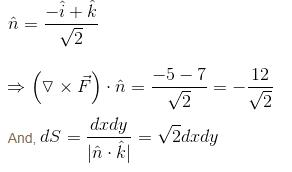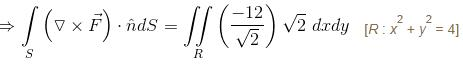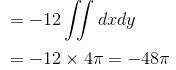Courses

# Vector Calculus MCQ Level - 1

## 10 Questions MCQ Test Basic Physics for IIT JAM | Vector Calculus MCQ Level - 1

Description
This mock test of Vector Calculus MCQ Level - 1 for Physics helps you for every Physics entrance exam. This contains 10 Multiple Choice Questions for Physics Vector Calculus MCQ Level - 1 (mcq) to study with solutions a complete question bank. The solved questions answers in this Vector Calculus MCQ Level - 1 quiz give you a good mix of easy questions and tough questions. Physics students definitely take this Vector Calculus MCQ Level - 1 exercise for a better result in the exam. You can find other Vector Calculus MCQ Level - 1 extra questions, long questions & short questions for Physics on EduRev as well by searching above.
QUESTION: 1

### The angle between the  x2 + y2 + z2 = 9  and  z = x2 + y2 – 3  at the point (2, –1, 2) is :

Solution:

The angle between the surface at point is the angle between the normal to the surface at the point.

A normal to x2 + y2 + z2 = 9 at (2, -1, 2) is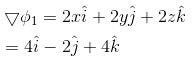A normal to z = x2 + y2 - 36 at (2, -1, 2) is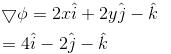We know that,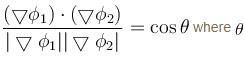is the required angle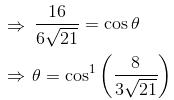The correct answer is: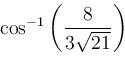QUESTION: 2

### For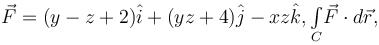where C is the square in xy–plane projected from the cube x = 0, x = 2, y = 0, y = 2, z = 0, z = 2 above xy–plane, will be equal to :

Solution:

By Stoke’s theorem,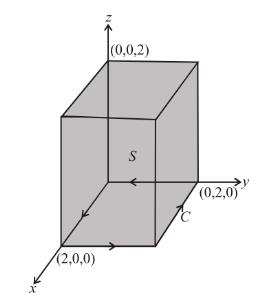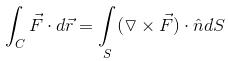∴  we need to evaluate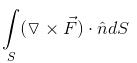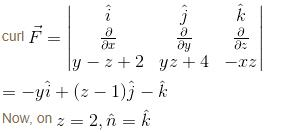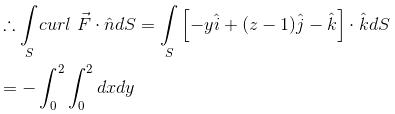= –4

QUESTION: 3

### If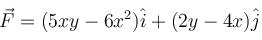and C is the curve  y = x3  from the point (1, 1) to (2, 8), then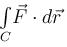will be :

Solution: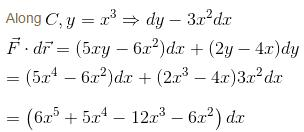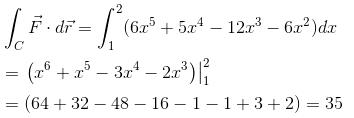QUESTION: 4

The value of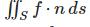where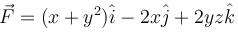and S in the surface of the plane 2x + y + 2z = 6 in the first octant will be

Solution: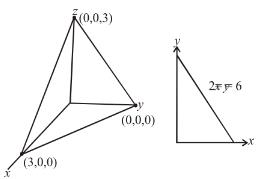Normal to the surface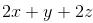= constant will be :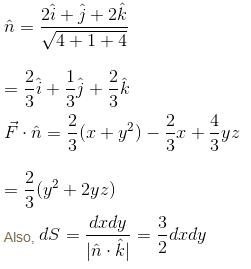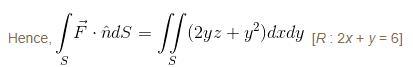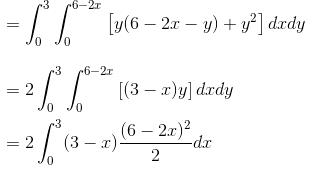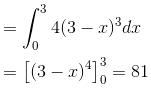QUESTION: 5

Using Gauss Divergence theorem, find the value of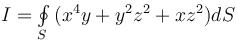over the entire surface of the sphere x2 + y2 + z2 = 1 equal to :

Solution:

Comparing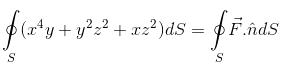where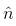(normal to the surface) =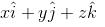we get,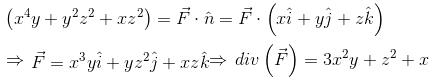By Gauss Divergence Theorem,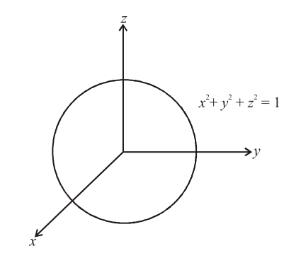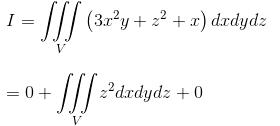[∵ 3x2y is an odd function of y and x is an odd function of x]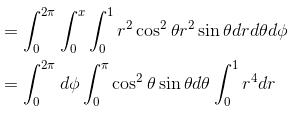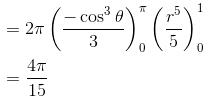The correct answer is: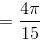QUESTION: 6

The value of the line integral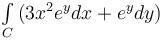where, C is the boundary of the region lying between the squares with vertices (1, 1), (–1, 1), (–1, –1) and (1, –1) and (2, 2), (–2, 2), (–2, –2) and (2, -2) will be :

Solution:

By Green’s Theorem,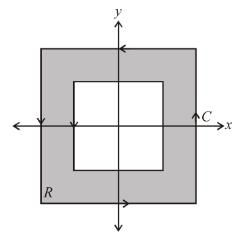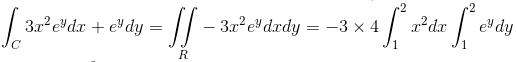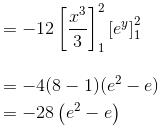The correct answer is: -28(e2 - e)

QUESTION: 7

The value of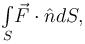where,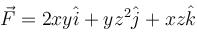and S is the surface of the parallelepiped bounded by x = 0, y = 0, z = 0, x = 2, y = 1, z = 3 will be :

Solution: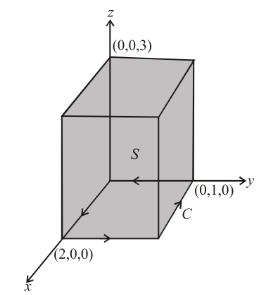By Gauss Divergence Theorem,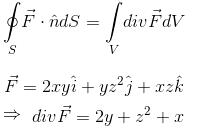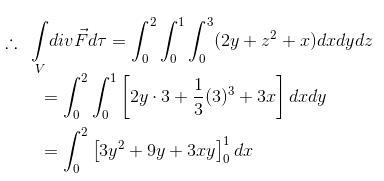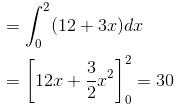QUESTION: 8

If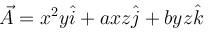and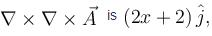then (a, b) =

Solution: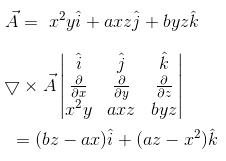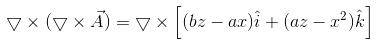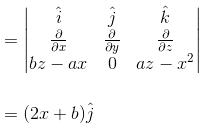which is given to be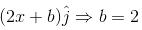Hence,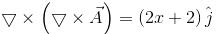for b = 2 and a being any value.

The correct answer is: (1, 2)

QUESTION: 9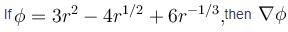is equal to :

Solution: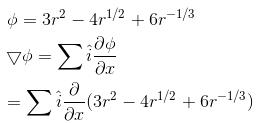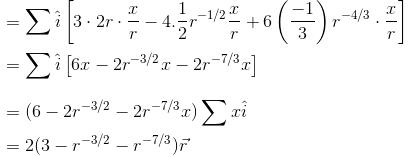The correct answer is: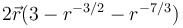QUESTION: 10

The value of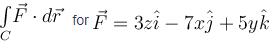where C is the intersection of  z = x + 4 with x2 + y2 = 4  will be :

Solution: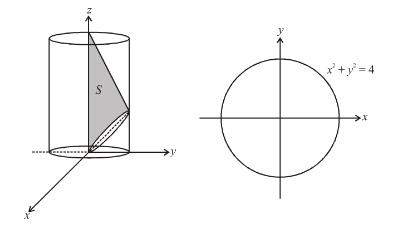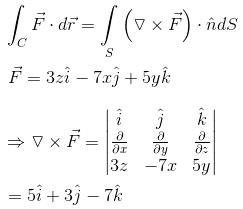Also, the normal to the surface z – x = constant is